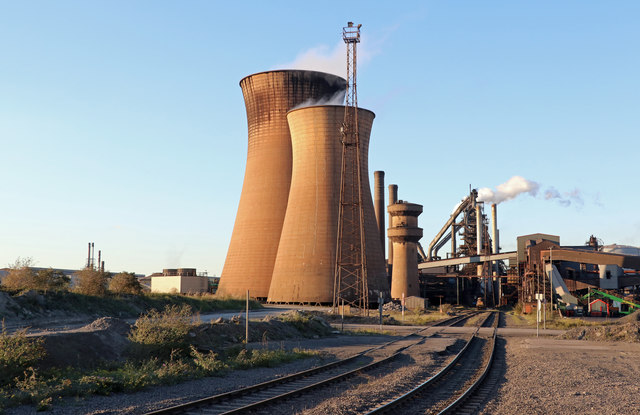Chemistry µGCSE:

Calculating Masses

10 quick questions - for GCSE and iGCSE

10 minutes maximum! (can you do it in 5?)

Grab a calculator and Periodic Table - time for some maths!

1. Lead(II)iodide is produced in a precipitation reaction:

Pb(NO3)2 (aq) +  2KI (aq)   PbI2 (s) + 2KNO3 (aq)

The amount, in moles, of each reactant needed to produce 0.30 moles of lead(II)iodide is:Moles of Pb(NO3)2 (aq) Moles of KI (aq) A 0.1 0.2 B 0.15 0.3 C 0.3 0.6 D 0.3 0.3
2. Powdered calcium carbonate reacts vigorously with dilute hydrochloric acid in the following reaction:

CaCO3 + 2HCl    CaCl2 + H2O + CO2

The maximum mass of carbon dioxide which could be produced from 0.2 moles of calcium carbonate is:
[Ar of C = 12; Ar of O = 16]

• A.   0.088g
• B.   0.2g
• C.   4.4g
• D.   8.8g
3. Iron reacts with excess sulfuric acid to give iron(II)sulfate.

The equation for the reaction is:

Fe + H2SO4 FeSO4 + H2

What mass of iron is needed to produce 7.6 g of iron(II)sulfate?
[Ar of Fe = 56;  Mr of FeSO4 =  152]

• A.   2.8g
• B.   5.6g
• C.   7.6g
• D.   20.6g
4. Magnesium burns in oxygen forming magnesium oxide.

The equation for the reaction is:

2Mg + O2 2MgO

The maximum mass of magnesium oxide formed from 2.4 g of magnesium is …..

• A.  2.0g
• B.  4.0g
• C.  6.0g
• D.  8.0g

Q5+6.
Iron is obtained by reducing iron(III)oxide in a blast furnace using the gas carbon  monoxide as the reducing agent.
The equation for reaction is:

Fe2O3 (s) + 3CO (g)  2Fe (s) + 3CO2 (g)5. The formula mass of iron(III) oxide and the number of moles of iron(III)oxide in 320kg is …

 Formula mass of Fe2O3 Number of moles of Fe2O3 in 320kg A 160 2 B 72 4.4 C 160 2000 D 72 4444
6. The mass of iron obtained from 320 kg of iron(III) oxide is…….
• A.   112 kg
• B.   224 kg
• C.   249 kg
• D.   498 kg

7. In an experiment 0.096g of magnesium is combined with 0.006 moles of hydrochloric acid and the following reaction takes place:

Mg + 2HCl MgCl2 + H2

The number of moles of magnesium used and the reagent in excess is:

 Number of moles of magnesium Reagent in excess A 0.004 magnesium B 0.004 Hydrochloric acid C 0.003 magnesium D 0.003 Hydrochloric acid
8. 7.8g of metal X reacted with 0.05 moles of oxygen gas in the following reaction:

4 X + O2   2 X2O.

Determine the number of moles of metal X which reacted and the relative atomic mass of metal X.

 Number of moles of metal X reacted Relative atomic mass of X A 0.1 39 B 0.1 78 C 0.2 39 D 0.2 78
9. Glucose is fermented to produce ethanol and carbon dioxide:

C6H12O6  2C2H5OH + 2CO2

90g of glucose was fermented. Calculate the number of moles of glucose fermented and the amount, in moles, of ethanol produced.
[Mr of glucose = 180]

 Number of moles of glucose fermented Moles of ethanol produced A 2 4 B 2 2 C 0.5 0.5 D 0.5 1

10. A 3.36g sample of sodium hydrogen carbonate, NaHCO3, was heated until it decomposed fully.

2.12g of sodium carbonate, Na2CO3, was produced.

What is the simplest whole number ratio, in moles, of sodium hydrogen carbonate decomposed to sodium carbonate formed?

[Mr of NaHCO3 is 84; Mr of Na2CO3 is 106]Ratio NaHCO3 : Na2CO3 A 1:2 B 2:1 C 3:2 D 4:2